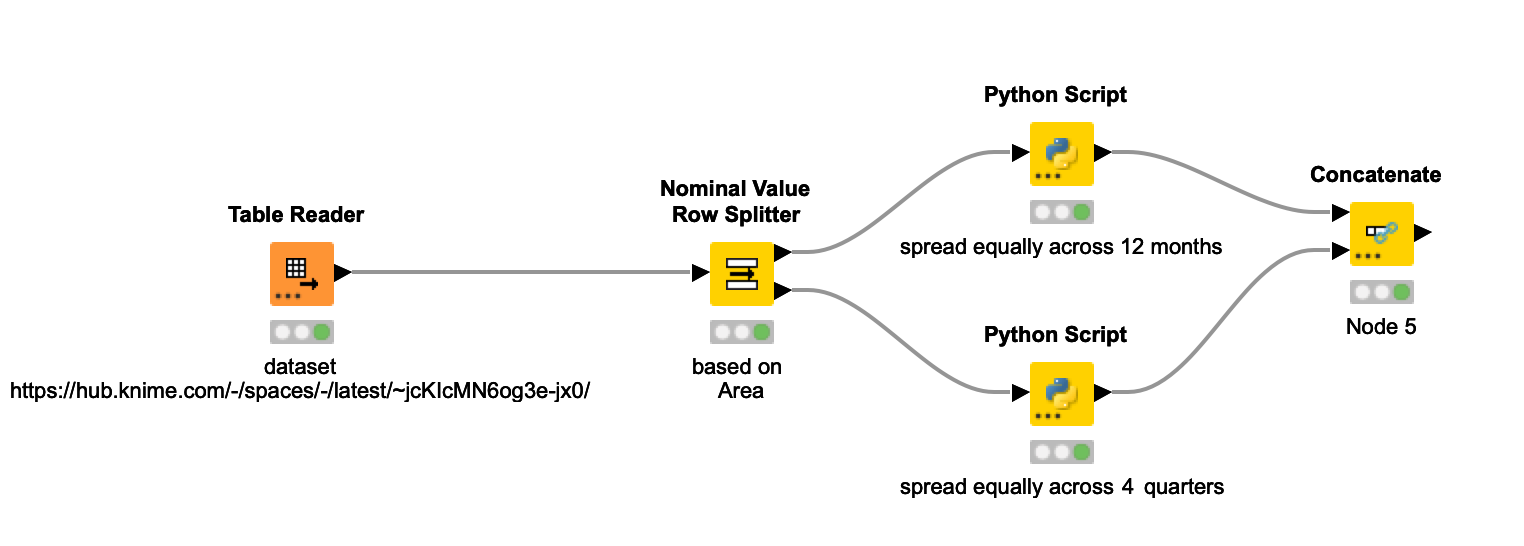# 🥷 [S2] Challenge 04

· 阅读需 1 分钟

Just KNIME It, Season 2 / Challenge 04 reference## Process​

Divide area A and area B first, then processed according to different time requirements through the Python node.

### spread equally across 12 months​

import knime.scripting.io as knioimport pandas as pddf = knio.input_tables.to_pandas()# Calculate the monthly expense allocationdf['exp'] /= 12# Duplicate the rows for each monthdf = df.loc[df.index.repeat(12)].reset_index(drop=True)df['time_range'] = pd.date_range(start='2022-01-01', periods=len(df), freq='MS').strftime('%b')knio.output_tables = knio.Table.from_pandas(df)

### spread equally across 4 quarters​

import knime.scripting.io as knioimport pandas as pddf = knio.input_tables.to_pandas()# Define the number of quartersnum_quarters = 4# Expand the table with a row for each quarterdf_expanded = pd.concat([df] * num_quarters, ignore_index=True)# Add a column for the quarter indexdf_expanded['time_range'] = ['Q{}'.format(i+1) for i in range(num_quarters)] * len(df)# Calculate the amount of exp for each quarter for each projectexp_per_quarter = df_expanded['exp'] / num_quarters# Add a column for the exp for each quarterdf_expanded['exp'] = exp_per_quarterknio.output_tables = knio.Table.from_pandas(df_expanded)

## Another thoughts​

BTW, this is just for practice. I do believe it is more easy to create a constant time_range table, then just use cross join node.